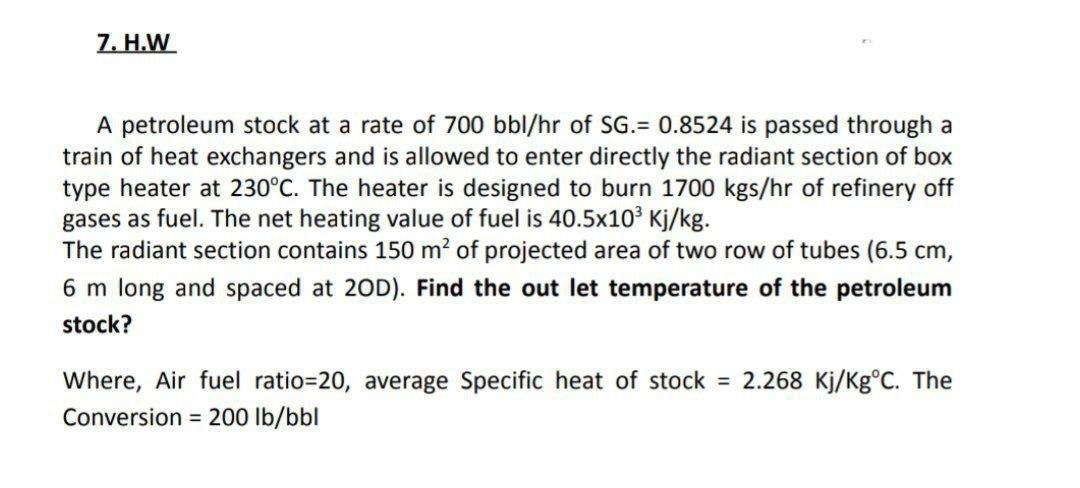# Question 7. H.W A petroleum stock at a rate of 700 bbl/hr of SG.= 0.8524 is passed through a train of heat exchangers and is allowed to enter directly the radiant section of box type heater at 230°C. The heater is designed to burn 1700 kgs/hr of refinery off gases as fuel. The net heating value of fuel is 40.5x103 Kj/kg. The radiant section contains 150 m2 of projected area of two row of tubes (6.5 cm, 6 m long and spaced at 20D). Find the out let temperature of the petroleum stock? Where, Air fuel ratio=20, average Specific heat of stock = 2.268 Kj/Kg°C. The Conversion = 200 lb/bblCE7GH0 The Asker · Chemical EngineeringTranscribed Image Text: 7. H.W A petroleum stock at a rate of 700 bbl/hr of SG.= 0.8524 is passed through a train of heat exchangers and is allowed to enter directly the radiant section of box type heater at 230°C. The heater is designed to burn 1700 kgs/hr of refinery off gases as fuel. The net heating value of fuel is 40.5x103 Kj/kg. The radiant section contains 150 m2 of projected area of two row of tubes (6.5 cm, 6 m long and spaced at 20D). Find the out let temperature of the petroleum stock? Where, Air fuel ratio=20, average Specific heat of stock = 2.268 Kj/Kg°C. The Conversion = 200 lb/bbl
More
Transcribed Image Text: 7. H.W A petroleum stock at a rate of 700 bbl/hr of SG.= 0.8524 is passed through a train of heat exchangers and is allowed to enter directly the radiant section of box type heater at 230°C. The heater is designed to burn 1700 kgs/hr of refinery off gases as fuel. The net heating value of fuel is 40.5x103 Kj/kg. The radiant section contains 150 m2 of projected area of two row of tubes (6.5 cm, 6 m long and spaced at 20D). Find the out let temperature of the petroleum stock? Where, Air fuel ratio=20, average Specific heat of stock = 2.268 Kj/Kg°C. The Conversion = 200 lb/bbl